Next: Topology Up: Lie Algebras Previous: Unitary Matrices

Picturing the Correspondences

We have an embarrassment of riches: so many correspondences that it is easy to get confused. This paragraph tries to fit things into a coherent framework, to make them easier to remember.

We have two fundamental concepts that are easy to visualize:

Rotations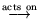vectors in 3-space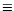angular velocities

The rotation group acts on the space of vectors. For any representation of the rotation group and any representation of the vector space, we would like to have an intuitive grasp of the action. (Since the action preserves distances, one can also consider the action of the group just on the set of vectors of norm 1, i.e., on the sphere.)

Possible representations for the rotation group: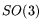,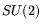, quaternions of norm 1. Possible representations for the vector space: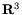,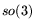,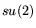, and the space of traceless Hermitian matrices. Forwith, the action is matrix multiplication on the left: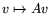. Forwith, orwithor the traceless Hermitian matrices, the action is conjugation:.

The actions withare all faithful. The actions withare all two-to-one: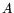and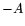determine the same action (i.e., rotation).

Let's look at theactions in more detail. The space of traceless Hermitian matrices consists of all matrices of the form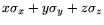,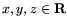. This is in one-one correspondence with: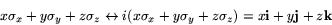So if we understand oneaction, we understand the other. I'll use Pauli matrices from now on.

An arbitary elementoflooks like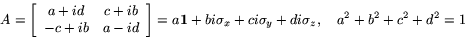and we see that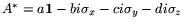. So the result of acting withon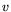can be computed simply by working out the product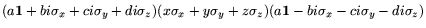. For this we need the multiplication table of the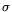's. This is simply: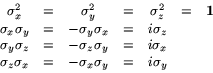The easiest way to check this is to work out the action of the's on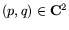: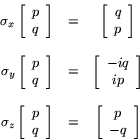We have here non-commuting Hermitian matrices whose product is not Hermitian-- in fact is anti-Hermitian. Now, one has an analogy between matrices and complex numbers, under which Hermitian'' goes with real'', anti-Hermitian'' goes with purely imaginary'', and unitary'' goes with on the unit circle''. Thematrices provide a striking example of the analogy breaking down. Non-commutativity is the culprit-- for of course the product of commuting Hermitian matrices is Hermitian.

Example: what rotation does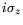represent? Its action on the unit x vector,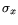, is just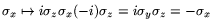. Similar calculations show that y goes to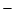y and z stays put, so we have a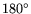rotation about the z-axis. Similarly for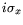and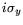.

The exponential map provides a mapping frominto: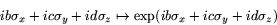(Warning: the exponential of a sum is not in general the product of the exponentials, because of non-commutativity.) For the rotation group (as we've seen) this says simply that an angular velocity determines a rotation-- e.g., by the prescription rotate at the given angular velocity for one time unit''. The basis of angular velocities'' inis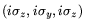. Let us consider rotations about the z-axis.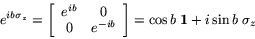(sinceacts separately on each coordinate.) Perhaps the clearest way to exhibit the action of this rotation on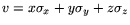is to work entirely in matrix form: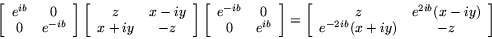i.e., a rotation about the z-axis of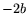radians. Exercise: the same sort of thing holds forand.

So one can directly picture the action ofon vectors in 3-space. The double angles''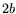, etc., stem from the two multiplications in the action: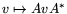. And the double angles in turn are the reason the map fromtois two-to-one.acts on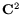via left multiplication:, whereis a column vector. Can one pictureas some kind of geometric object in 3-space? Yes indeed! An object known as a spin vector embodiesgeometrically. But I won't get to them.Next: Topology Up: Lie Algebras Previous: Unitary Matrices